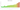# S/.

## Peruvian Nuevo Sol

### Peruvian sol

#### how has the value of the currency changed in the last year?## USD 1 = PEN 4.108

How much is10 US dollars worth inPeruvian soles?
At the current exchange rate, 10 US dollars is worth 41.08 Peruvian soles
How much is50 US dollars worth inPeruvian soles?
At the current exchange rate, 50 US dollars is worth 205.4 Peruvian soles
How much is100 US dollars worth inPeruvian soles?
At the current exchange rate, 100 US dollars is worth 410.8 Peruvian soles
How much is500 US dollars worth inPeruvian soles?
At the current exchange rate, 500 US dollars is worth 2,054 Peruvian soles
How much is2,000 US dollars worth inPeruvian soles?
At the current exchange rate, 2,000 US dollars is worth 8,216 Peruvian soles
How much is10 US dollars worth inPeruvian soles?
At the current exchange rate, 10 US dollars is worth 41.08 Peruvian soles
How much is50 US dollars worth inPeruvian soles?
At the current exchange rate, 50 US dollars is worth 205.4 Peruvian soles
How much is100 US dollars worth inPeruvian soles?
At the current exchange rate, 100 US dollars is worth 410.8 Peruvian soles
How much is500 US dollars worth inPeruvian soles?
At the current exchange rate, 500 US dollars is worth 2,054 Peruvian soles
How much is2,000 US dollars worth inPeruvian soles?
At the current exchange rate, 2,000 US dollars is worth 8,216 Peruvian soles